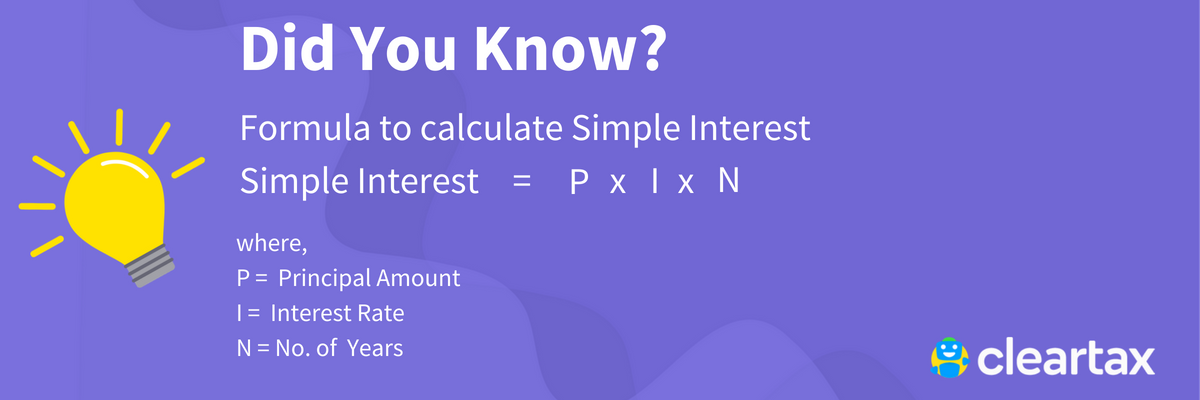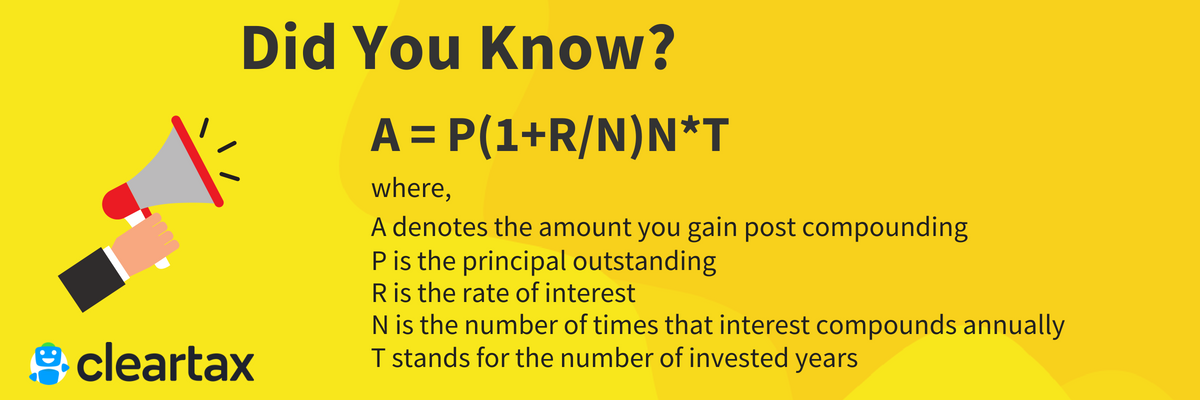# Invest in Mutual Funds & Get More Returns than Bank Deposit

Whether you lend to or borrow from a financial institute or a company, it has a price tag attached to it. And you know that price by the name of interest. The cost is often borne by the one who borrows as per agreement from both the parties. There are two ways to calculate interest – simple interest and compound interest. They form the basis of all financial dealings, and it is important for you as an investor to become acquainted with the concept to make informed financial decisions. In this article, we will discuss simple and compound interest in detail and how financial institutes employ them.

• Simple Interest
• Compound Interest
• Compounding Periods
• Simple Interest Vs Compound Interest
• Power of Compounding
• ## 1. Simple Interest

Here, the lender levies interest only on the amount he has loaned. The former is the type of interest where the interest is charged only on amount loaned originally. So, simple interest is the sum paid for using the borrowed money, for a fixed period. It is essentially a percentage of the actual loan charged for the borrowing tenure. For instance, when you put an FD of Rs. 1 lakh in a bank at the rate of 7% for one year, the bank uses your money for its operations in that duration. In exchange for that you will receive Rs. 7000 interest income annually.

See how easy and quick it is to estimate simple interest! Auto loan is another example for this. The more money you borrow/lend and the longer the tenure is, the greater will be the interest.Here is how you calculate simple interest.## 2. Compound Interest

Compound interest means the money you make other than simple interest income from your invested capital. So, your investment grows dramatically thanks to the power of compounding. Basically, it gives you potential to earn more than regular simple interest on your capital. It is up to the bank (or financial institute) to decide how often the interest can be compounded – daily, monthly, quarterly, six-monthly or annually. With more frequency, your interest accrual will also increase.

Of course, compound interest is highly beneficial as the pace of wealth growth is more here. For instance, if you invest Rs. 5000 at the rate of 10% interest and it compounds annually for 3 years, your corpus (investment + gains) will be Rs. 6655. Your gains will be Rs. 1655 as opposed to the Rs. 1500 you will make in simple interest. Again, if you increase the frequency of compounding from once a year to more, the interest income will be even higher. Therefore, finding out the compounding frequency can help you make a more lucrative choice when it comes to investing.

Here is the formula to get accurate compound interest:## 3. Compounding Periods

To estimate compound interest, it is important to take into account the number of compounding periods. They can make a huge (positive) difference to the accrued money. As mentioned above, with more number of compounding periods in the investment tenure, the corpus amount also increases accordingly. This is because interest is calculated at the end of each period. It could be on a monthly or weekly or quarterly or annual basis. For instance, if you invest Rs. 100,000 for 5 years and compounds every year, you will earn more compared to having it compounded less than that.

## 4. Simple Interest Vs Compound Interest

 Simple Interest Compound Interest Levied only on the loan amount or principal Levied on the loan amount as well as its interest A small percentage of the principal as agreed between lender and borrower A small percentage of principal and amassed simple interest as mutually agreed Steady wealth growth Wealth growth increases at a higher pace due to compounding Less returns compared to compound interest Higher returns compared to simple interest Wealth growth is comparatively lower Wealth accumulation will be at a higher rate Principal never changes with increased tenure Principal increases as interest compounds and gets added to it Easy to calculate using the formula P*I*N Difficult to calculate using the formula P(1+R/N)N*T

## 5. Power of Compounding

To tap the power of compounding, you need to invest in any scheme that follows compound interest system like mutual fund or saving scheme, and then let it be. As they generate interest or dividends, the profits get reinvested and compounds the earnings at a fast-tracked rate. You can easily use our online calculators to estimate your earnings after required tenure.

Here, interest rates can make a huge difference. If the investment is more on the risky side, scope for higher gains also increases. Example, a savings account gives less interest, but investing in a mutual fund can earn you supreme returns over a longer tenure. Compounding is highly recommended because it can beat inflation. For instance, if your capital is making 3% interest and the inflation rate is 4%, you will face a 1% loss.

In short, it is not just important to invest, but also to explore ways to get the most out of it. Cleartax Invest has handpicked top-performing funds from the best fund houses in the country for you. You can choose investments based on your affordability and risk profile. So, don’t delay anymore and start investing.